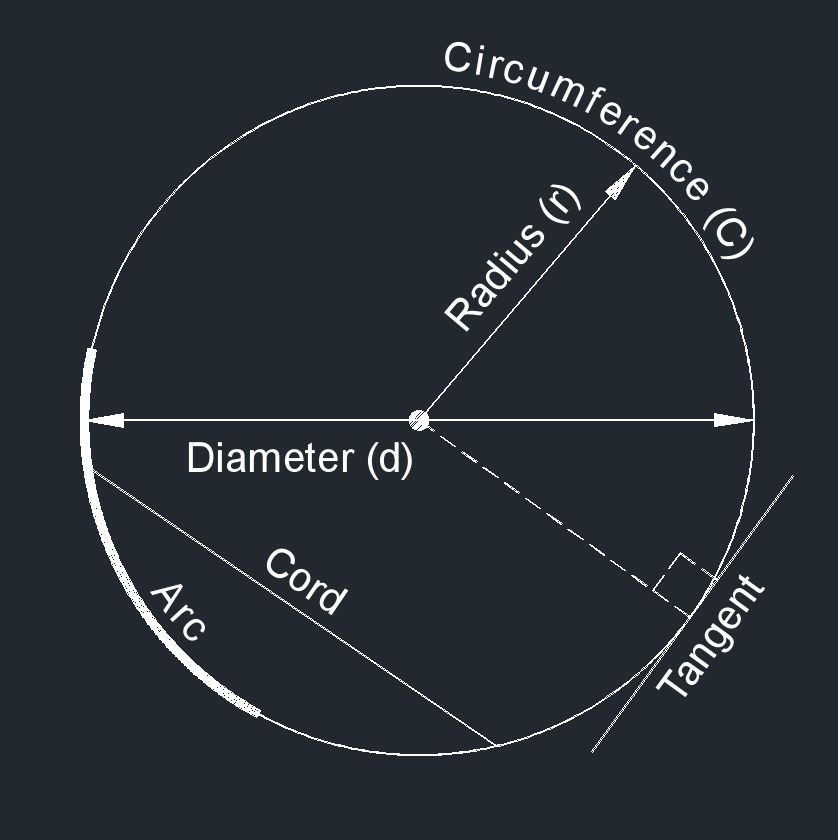# Diameter of a Circle

Written by Jerry Ratzlaff on . Posted in Plane GeometryIn geometry, a diameter of a circle is any straight line segment that passes through the center of the circle and whose endpoints are on the circle. The diameters are the longest chords of the circle.

In the process industry, the diameter is typically used to describe the size pipe that the process is flowing through. Unless explictily specified, the diameter is assumed to mean the nominal pipe size (NPS). The inside diameter of a pipe is the longest distance between the two inside walls of the pipe. The outside diameter is the distance between the two outside walls. To find the thickness of the pipe, subtract the outside diameter from the inside diameter and divide by two.

When sizing flow meters or impact tees, a certain straight run maybe required. This is typically specified in terms of diameters. For example a 10" orifice meter with a 10 diameter upstream requirement will require 100" of unobstructed straight run upstream of the orifice plate.

### Diameter of a Circle formula

$$\large{ d = 2 \; r }$$

$$\large{ d = \frac {C} {\pi} }$$

$$\large{ d = \sqrt {\frac {4 \; A} {\pi} } }$$

Where:

$$\large{ d }$$ = diameter

$$\large{ A }$$ = area

$$\large{ C }$$ = circumference

$$\large{ r }$$ = radius

$$\large{ \pi }$$ = Pi